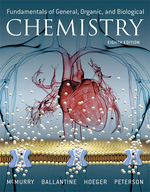×
Get Full Access to Fundamentals Of General, Organic, And Biological Chemistry (Mastering Chemistry) - 8 Edition - Chapter 1 - Problem 1.40
Get Full Access to Fundamentals Of General, Organic, And Biological Chemistry (Mastering Chemistry) - 8 Edition - Chapter 1 - Problem 1.40

×ISBN: 9780134015187 2044

## Solution for problem 1.40 Chapter 1

Fundamentals of General, Organic, and Biological Chemistry (Mastering Chemistry) | 8th Edition

• Textbook Solutions
• 2901 Step-by-step solutions solved by professors and subject experts
• Get 24/7 help from StudySoup virtual teaching assistantsFundamentals of General, Organic, and Biological Chemistry (Mastering Chemistry) | 8th Edition

4 5 1 390 Reviews
23
4
Problem 1.40

Butane $$\left(\mathrm{C}_{4} \mathrm{H}_{8}\right)$$ is an easily compressible gas used in cigarette lighters. It has a melting point of $$-138.4 { }^{\circ} \mathrm{C}$$ and a boiling point of $$-0.5{ }^{\circ} \mathrm{C}$$. Would you expect a butane lighter to work in winter when the temperature outdoors is 269 K? Why or why not? (Refer to Figure 1.9.)

Text Transcription:

C_4H_8

-138.4^circ C

–0.^circ C

Step-by-Step Solution:
Step 1 of 3

Molly Kitchen CHM1050 09-09-2015 Class Lecture Molecule: at least two atoms held in a definite arrangement by chemical bonds Imperial formula: lowest whole number ratio of the atoms Naming Compounds- AKA "nomenclature" Ionic compounds = metal (+charged/ aution) + nonmetal (-charged/ anion) BINARY (2 types of atoms) name: "metal" + "base of anion"+ "ide" NaCl = Sodium Chloride KBr = potassium bromide ZnI2= Zinc Mg (+2) Cl (-) = MgCl 2 Ternary (3 types of atoms) Compounds: Na(OH) sodium hydroxide Ca(OH) calcium hydroxide 2+ + Fe = ferrous FeCl2= ferrous chloride Cu = cuprous Fe3+ = ferric FeCl = ferric chloride Cu 2+= cupric 3 Stock System 2+ Mn Mn (II) "manganese two" Mn 3+ Mn (III)"manganese three" 4+ Mn Mn (IV)"manganese four" Naming Molecular Compounds "name of 1st atom" + "base of 2nd atom" + "ide" SiC = silicon carbide CO = carbon monoxide CO2= carbon dioxide HCl = hydrogen chloride Molly Kitchen CHM1050 Acid: something that yields hydrogen when added to water

Step 2 of 3

Step 3 of 3

##### ISBN: 9780134015187

Unlock Textbook Solution